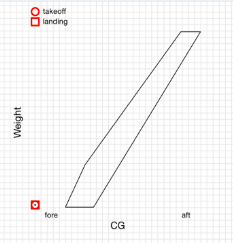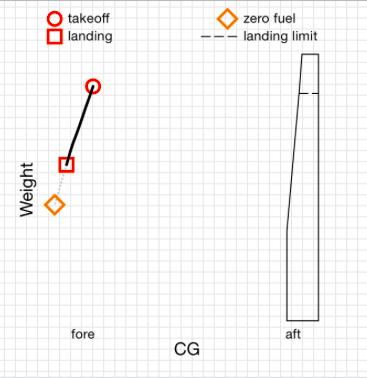# Why can't I get my CG into the CG envelope?

Is your CG Envelope slanted as pictured below, or is the CG not in the envelope?Probable CG Envelope data problem

If yes, check that the numbers entered to define the CG Envelope are Arms and not Divided Moments. Some Pilot's Operating Handbooks (POH) have a CG Envelope that displays a CG envelope with a Moment number that has been divided by 100 or 1000. This will make the numbers look potentially like an Arm.

If this is the case, take the Moment number listed and multiply it by the number the document claims the Moment was divided by. Once you have done that, divide that number by the corresponding weight to get the Arm.

For example, if a Moment was divided by 1000, take the displayed number (23.5) and multiply by 1000. Next, divide that number by the corresponding weight (1650 lbs). Formula:  (23.5x1000)/1650 = 14.24  -OR-  Total Moment/Weight=Arm

If your CG envelope looks very narrow and off to the right as pictured below, then view the following article:

Why is the Center of Gravity envelope distorted or squished off to the right side, and it shows out of CG?Probable Empty Weight data problem